## Roman Coefficient

A generalization of the Binomial Coefficient whose Notation was suggested by Knuth,(1)

The above expression is read Romanchoose.'' Whenever the Binomial Coefficient is defined (i.e.,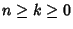or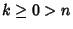), the Roman coefficient agrees with it. However, the Roman coefficients are defined for values for which the Binomial Coefficients are not, e.g.,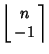(2)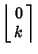(3)

where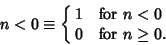(4)

The Roman coefficients also satisfy properties like those of the Binomial Coefficient,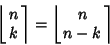(5)(6)

an analog of Pascal's Formula(7)

and a curious rotation/reflection law due to Knuth(8)

(Roman 1992).

Roman, S. The Logarithmic Binomial Formula.'' Amer. Math. Monthly 99, 641-648, 1992.